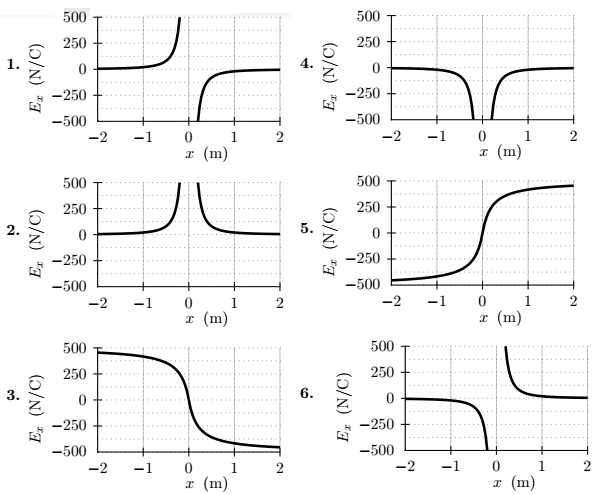# Problem: A charge of 3 μC is at the origin. Sketch the function E x versus x for both positive and negative values of x. (Remember that Ex is negative when E points in the negative x direction.)

###### FREE Expert Solution
86% (197 ratings)
###### Problem Details

A charge of 3 μC is at the origin. Sketch the function E x versus x for both positive and negative values of x. (Remember that Ex is negative when E points in the negative x direction.)Frequently Asked Questions

What scientific concept do you need to know in order to solve this problem?

Our tutors have indicated that to solve this problem you will need to apply the Electric Field concept. You can view video lessons to learn Electric Field. Or if you need more Electric Field practice, you can also practice Electric Field practice problems.

What professor is this problem relevant for?

Based on our data, we think this problem is relevant for Professor Adams' class at AU.